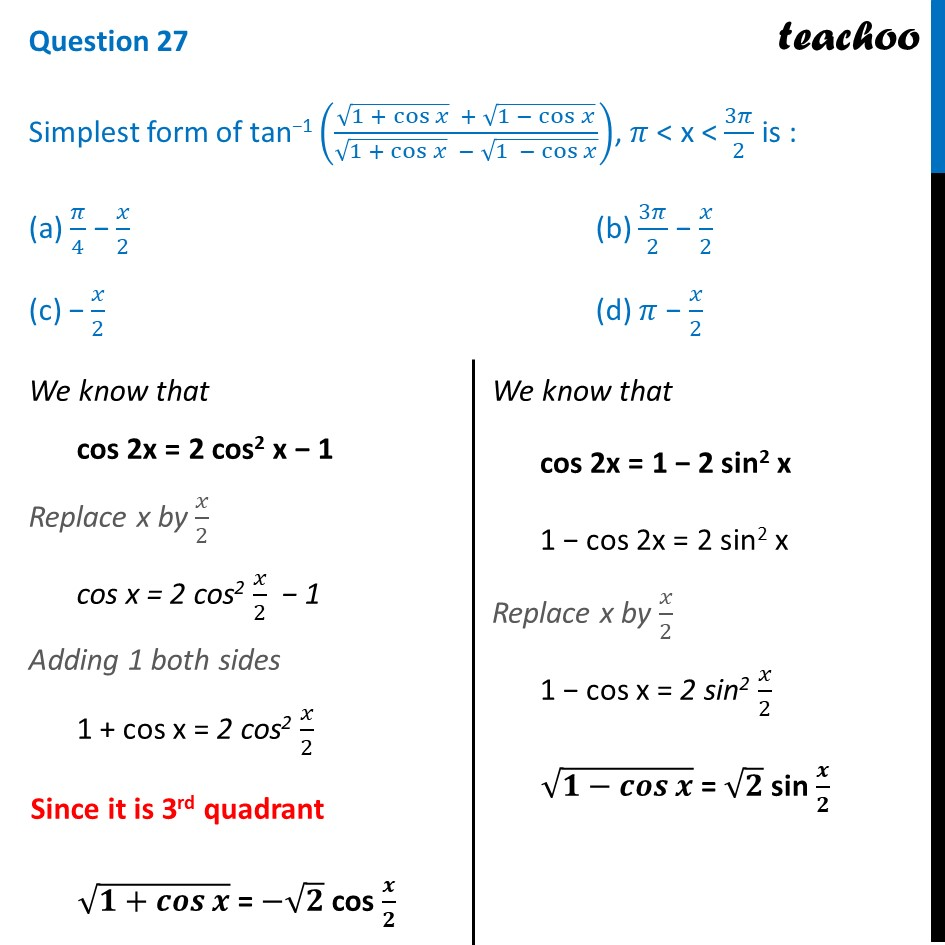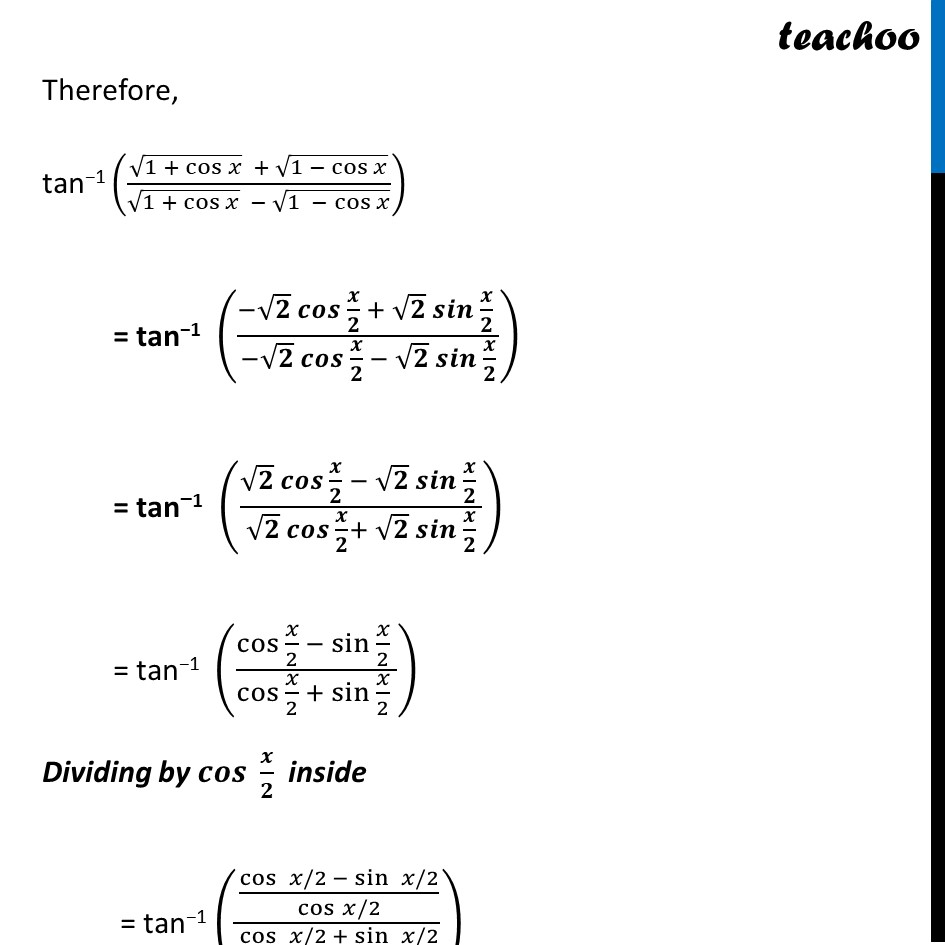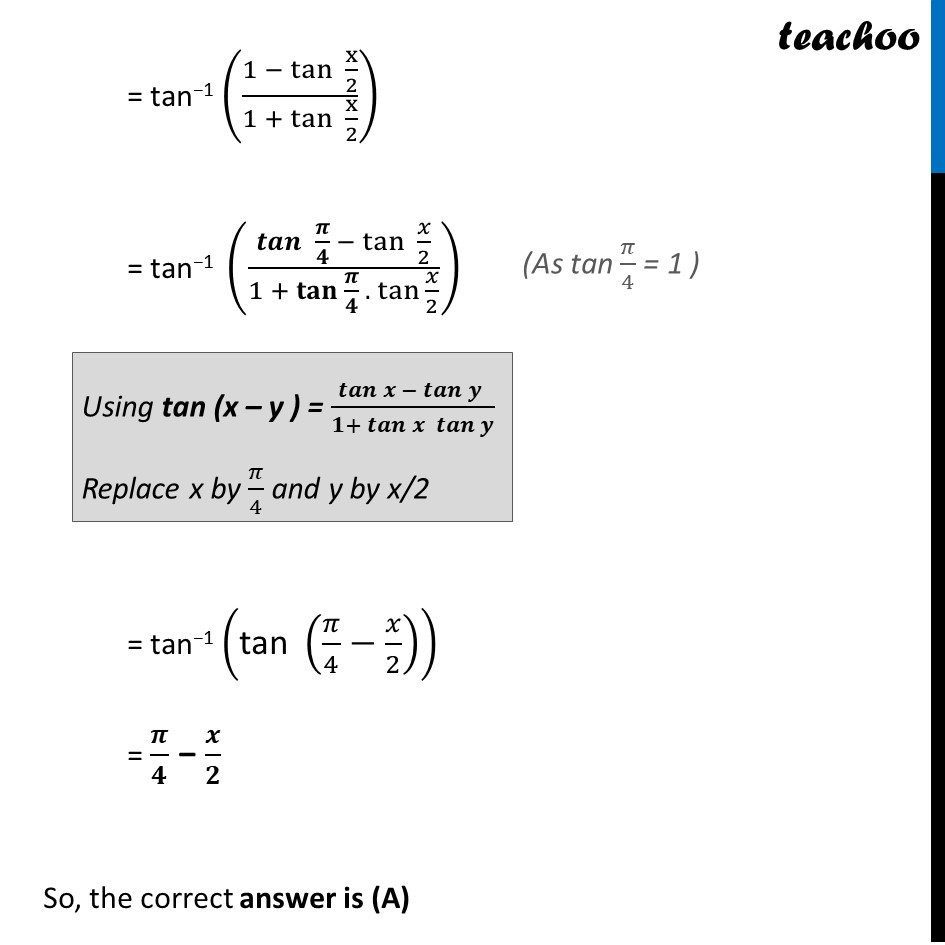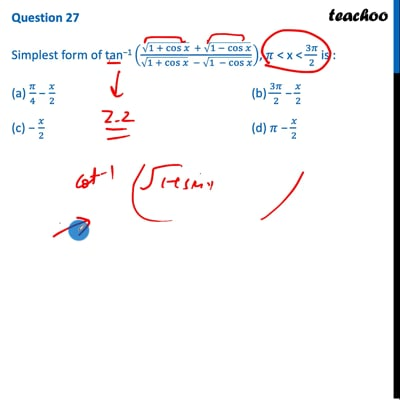CBSE Class 12 Sample Paper for 2022 Boards (MCQ Based - for Term 1)

Class 12
Solutions of Sample Papers and Past Year Papers - for Class 12 Boards

## (a) π/4 − x/2                (b) 3π/2 − x/2    (c) − x/2                       (d) π − x/2This video is only available for Teachoo black users

Get live Maths 1-on-1 Classs - Class 6 to 12

### Transcript

Question 27 Simplest form of tan−1 ((√(1 +〖 cos〗⁡𝑥 ) + √(1 − cos⁡𝑥 ))/(√(1 + cos⁡𝑥 ) − √(1 − cos⁡𝑥 ))), 𝜋 < x < 3𝜋/2 is : (a) 𝜋/4 − 𝑥/2 (b) 3𝜋/2 − 𝑥/2 (c) − 𝑥/2 (d) 𝜋 − 𝑥/2 We know that cos 2x = 2 cos2 x − 1 Replace x by 𝑥/2 cos x = 2 cos2 𝑥/2 − 1 Adding 1 both sides 1 + cos x = 2 cos2 𝑥/2 √(𝟏+𝒄𝒐𝒔⁡𝒙 ) = √𝟐 cos 𝒙/𝟐 We know that cos 2x = 1 − 2 sin2 x 1 − cos 2x = 2 sin2 x Replace x by 𝑥/2 1 − cos x = 2 sin2 𝑥/2 √(𝟏−𝒄𝒐𝒔⁡𝒙 ) = √𝟐 sin 𝒙/𝟐 Therefore, tan−1 ((√(1 +〖 cos〗⁡𝑥 ) + √(1 − cos⁡𝑥 ))/(√(1 + cos⁡𝑥 ) − √(1 − cos⁡𝑥 ))) = tan−1 ((√𝟐 〖𝒄𝒐𝒔 〗⁡〖𝒙/𝟐〗 + √𝟐 〖𝒔𝒊𝒏 〗⁡〖𝒙/𝟐〗 )/(√𝟐 〖𝒄𝒐𝒔 〗⁡〖𝒙/𝟐〗 − √𝟐 〖𝒔𝒊𝒏 〗⁡〖𝒙/𝟐〗 )) = tan−1 ((〖cos 〗⁡〖𝑥/2〗 − 〖sin 〗⁡〖𝑥/2〗 )/(〖cos 〗⁡〖𝑥/2〗 + 〖sin 〗⁡〖𝑥/2〗 )) Dividing by 〖𝒄𝒐𝒔 〗⁡〖𝒙/𝟐〗 inside = tan−1 (((〖cos 〗⁡〖𝑥/2〗 − 〖sin 〗⁡〖𝑥/2〗)/(cos⁡𝑥/2))/((〖cos 〗⁡〖𝑥/2〗 + 〖sin 〗⁡〖𝑥/2〗)/(cos⁡𝑥/2))) = tan−1 ((1 − tan⁡〖 x/2〗)/(1 +〖 tan〗⁡〖 x/2〗 )) = tan−1 ((𝒕𝒂𝒏⁡〖 𝝅/𝟒〗 − tan⁡〖 𝑥/2〗)/(1 + 〖𝐭𝐚𝐧 〗⁡〖𝝅/𝟒 .〖 tan 〗⁡〖𝑥/2〗 〗 )) = tan−1 ("tan " (𝜋/4−𝑥/2)) = 𝝅/𝟒 − 𝒙/𝟐 So, the correct answer is (A)